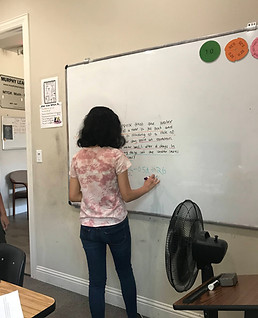top of page• # Each class is grouped by grade level• # Questions are designed to bridge the gap between what a student considers familiar to what is unfamiliar to them• # Topics are discussed and revisited every year## CONCEPTUAL LEARNING OF THE AREA OF A TRIANGLE

INSTRUCTOR: How do you find the area of a rectangle?

STUDENT: length x width

INSTRUCTOR: What is the area of this rectangle?

STUDENT: (5 x 10) = 50

INSTRUCTOR: Correct! Let's say I cut this this rectangle in half diagonally.

INSTRUCTOR: How big is the shaded region?

STUDENT: 50 ÷ 2 = 25

INSTRUCTOR: Right! And what shape is the shaded region?

STUDENT: A triangle!

INSTRUCTOR: So what would the area of a triangle be?

STUDENT: (b x h) ÷ 2

Instead of giving the student the answer directly, our instructors guide the students to the answer to maximize their understanding of the concepts. In this example, the area of a triangle is not provided to the student directly, but the instructor guides the student to the correct formula using the student's prior knowledge.

5

10

5

10

bottom of page